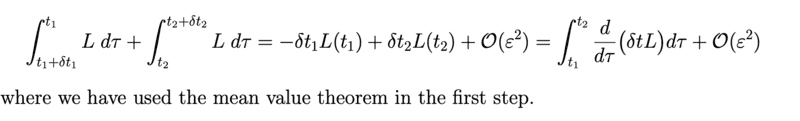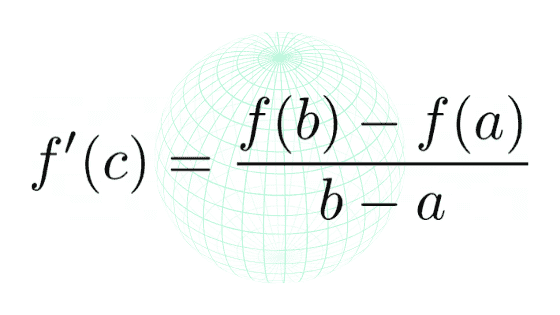# Noether's theorem time invariance -- mean value theorem use?

• A
Tush19how does the first step use mean value theorem? I don't get it , can anyone explain , thanks.

Staff Emeritus
Homework Helper
Gold Member
The mean value theorem states that
$$\int_x^{x+\delta x} f(s) ds = \delta x\, f(x^*)$$
where ##x \leq x^* \leq x + \delta x##. Since ##f## is continuous, ##f(x^*) \to f(x)## for small ##\delta x##.

•Tush19 and vanhees71
Tush19
The mean value theorem states that
$$\int_x^{x+\delta x} f(s) ds = \delta x\, f(x^*)$$
where ##x \leq x^* \leq x + \delta x##. Since ##f## is continuous, ##f(x^*) \to f(x)## for small ##\delta x##.
thanks but I couldn't find that mean value theorem statement anywhere ,all it shows that mean value theorem is the followingMentor
•Tush19 and vanhees71
Staff Emeritus
Homework Helper
Gold Member
•Tush19 and vanhees71
Staff Emeritus
Homework Helper
Gold Member
Just to add: The mean value theorem for definite integrals is easy to obtain from the theorem you quoted. Just consider that
$$(b-a) f’(c) = f(b) - f(a) = \int_a^b f’(x) dx$$
and let ##g(x) = f’(x)##. You now have
$$\int_a^b g(x) dx = (b-a) g(c)$$
for some ##c## such that ##a\leq c\leq b##.

•DrClaude, Tush19 and vanhees71
Tush19
Just to add: The mean value theorem for definite integrals is easy to obtain from the theorem you quoted. Just consider that
$$(b-a) f’(c) = f(b) - f(a) = \int_a^b f’(x) dx$$
and let ##g(x) = f’(x)##. You now have
$$\int_a^b g(x) dx = (b-a) g(c)$$
for some ##c## such that ##a\leq c\leq b##.
thank you so much